### 7. LUMINOSITY DISTANCE

The luminosity distance DL is defined by the relationship between bolometric (ie, integrated over all frequencies) flux S and bolometric luminosity L: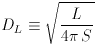(19)

It turns out that this is related to the transverse comoving distance and angular diameter distance by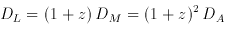(20)

(Weinberg 1972, pp. 420-424; Weedman 1986, pp. 60-62). The latter relation follows from the fact that the surface brightness of a receding object is reduced by a factor (1 + z)-4, and the angular area goes down as DA-2. The luminosity distance is plotted in Figure 3.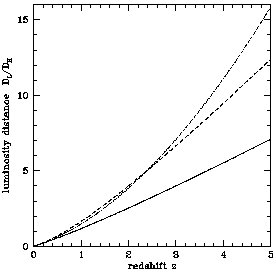Figure 3. The dimensionless luminosity distance DL / DH. The three curves are for the three world models, (M,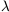) = (1, 0), solid; (0.05, 0), dotted; and (0.2, 0.8), dashed.

If the concern is not with bolometric quantities but rather with differential flux S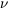and luminosity L, as is usually the case in astronomy, then a correction, the k-correction, must be applied to the flux or luminosity because the redshifted object is emitting flux in a different band than that in which you are observing. The k-correction depends on the spectrum of the object in question, and is unnecessary only if the object has spectrumL= constant. For any other spectrum the differential flux Sis related to the differential luminosity Lby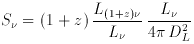(21)

where z is the redshift, the ratio of luminosities equalizes the difference in flux between the observed and emitted bands, and the factor of (1 + z) accounts for the redshifting of the bandwidth. Similarly, for differential flux per unit wavelength,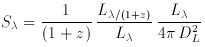(22)

(Peebles 1993, pp. 330-331; Weedman 1986, pp. 60-62). In this author's opinion, the most natural flux unit is differential flux per unit log frequency or log wavelengthS=Sfor which there is no redshifting of the bandpass so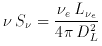(23)

wheree= (1 + z)is the emitted frequency.

The distance modulus DM is defined by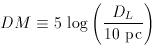(24)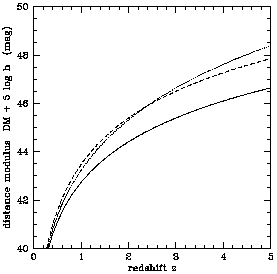Figure 4. The distance modulus DM. The three curves are for the three world models, (M,) = (1, 0), solid; (0.05, 0) dotted; and (0.2, 0.8) dashed.

because it is the magnitude difference between an object's observed bolometric flux and what it would be if it were at 10 pc (don't ask me, ask an astronomer!). The distance modulus is plotted in Figure 4. The absolute magnitude M is the astronomer's measure of luminosity, defined to be the apparent magnitude the object in question would have if it were at 10 pc, so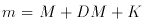(25)

where K is the k-correction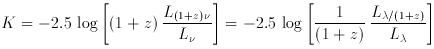(26)

(eg., Oke & Sandage 1968).